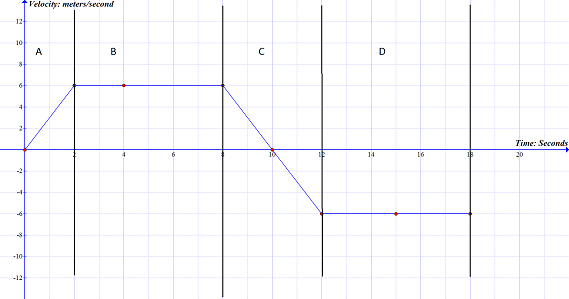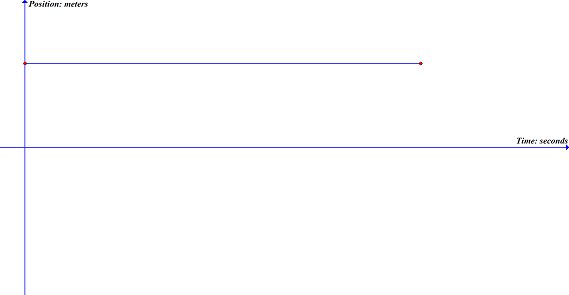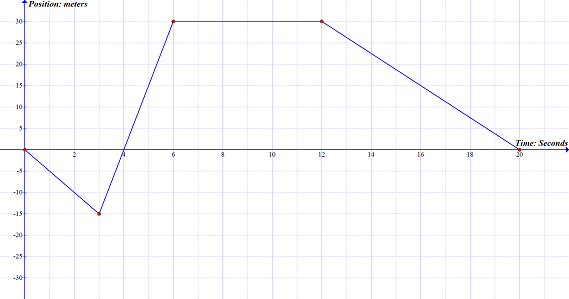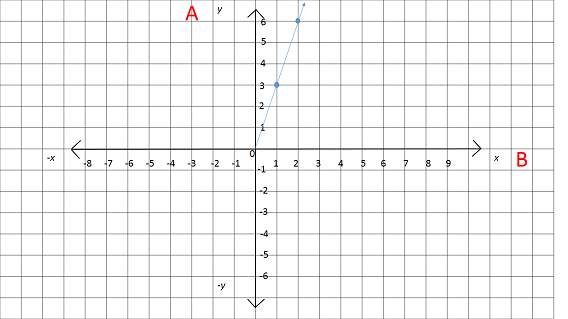# Kinematics Principles Chapter Exam

Exam Instructions:

Choose your answers to the questions and click 'Next' to see the next set of questions. You can skip questions if you would like and come back to them later with the yellow "Go To First Skipped Question" button. When you have completed the practice exam, a green submit button will appear. Click it to see your results. Good luck!

### Page 1

#### Question 4 4. Using the graph, calculate the displacement for the section labeled D.### Page 2

#### Question 6 6. This graph represents an object that is#### Question 7 7. For this graph, calculate the total average velocity.#### Question 8 8. Using the graph, calculate the displacement for the section labeled B.#### Question 9 9. This graph represents how fast you jogged over a specific period of time. If you traveled 3 meters in 1 second, using the graph, what label should be at position A?### Page 4

#### Question 16 16. For this graph, calculate the total average speed.### Page 6

#### Question 29 29. Using the graph, calculate the displacement for the section labeled A.#### Kinematics Principles Chapter Exam Instructions

Choose your answers to the questions and click 'Next' to see the next set of questions. You can skip questions if you would like and come back to them later with the yellow "Go To First Skipped Question" button. When you have completed the practice exam, a green submit button will appear. Click it to see your results. Good luck!

Support